## RC Driving Point Impedance function:

As the name indicates, the RC networks consist of only R and C components. There is no inductor in RC networks. The RC Driving Point Impedance function is denoted as ZRC(s). The properties of RC Driving Point Impedance function and the properties of driving point admittance function of RL network are identical. Thus the properties ZRC(s) and YRL(s) are same. There are no complex poles in RC network function. The poles and zeros alternate each other and are located in left half of s plane. To understand the properties of RC network function consider a driving point impedance function of RC network as,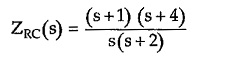The poles are at s = 0, – 2

The zeros are at s = –1, – 4

The pole-zero plot is shown in the Fig. 7.11.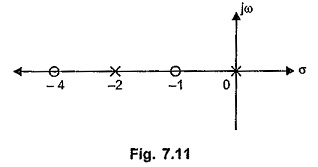#### Properties of RC Driving Point Impedance Function:

Referring to the pole zero plot of ZRC(s) function considered, the various properties of RC Driving Point Impedance function can be stated as,

1. The poles and zeros are simple. There are no multiple poles and zeros.
2. The poles and zeros are located on negative real axis.
3. The poles and zeros interlace (alternate) each other on the negative real axis.
4. We know that the poles and zeros are called critical frequencies of the The critical frequency nearest to the origin is always a pole. This may be located at the origin.
5. The critical frequency at a greatest distance away from the origin is always a zero, which may be located at ∞ also.
6. The partial fraction expansion of ZRC(s) gives the residues which are always real and positive.
7. There is no pole located at infinity.
8. The slope of the graph of Z(σ) against σ is always negative.
9. There is no zero at the origin.
10. The value of ZRC(s) at s=0 is always greater than the value of ZRC(s) at s = ∞.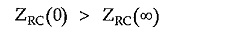It can, be seen that for a ZRC considered ZRC(0) = ∞ while ZRC(∞) = 1.

Consider a simple RC network as shown in the Fig. 7.12.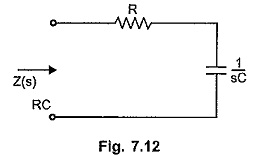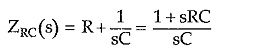Let us plot ZRC(σ) against σ where s = σ.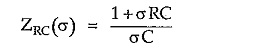To find the slope of ZRC(σ) against σ find d ZRC(σ)/dσ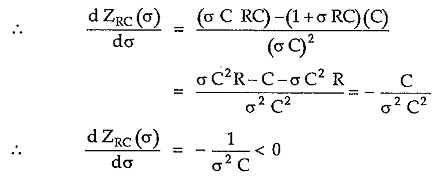Thus the slope of ZRC(σ) against σ is always negative for any value of σ.

The graph of Z(σ) against σ for the RC network function is shown in the Fig. 7.13.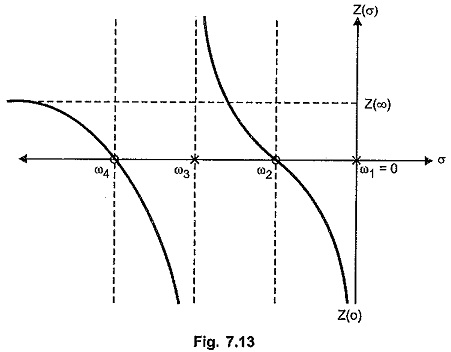ω123 and ω4 are the critical frequencies. At the critical frequencies like ω3, the Z(σ) changes its sign suddenly such that the slope always remains negative. The value of Z (∞) is constant so graph runs parallel to the σ axis, finally.

The nature of Z (σ) against σ graph when there is no pole at the origin is shown in the Fig. 7.14.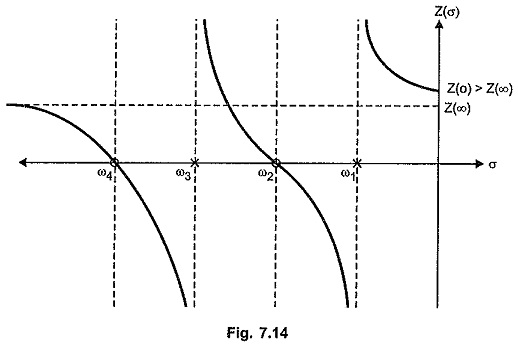All the properties of driving point admittance function of RL network [YRL(s)] are exactly identical to the properties of driving point impedance function of RC network [ZRC(s)].

#### Realization of Impedance Function of RC Network:

As mentioned earlier, the realization of ZRC(s) function can be achieved using Foster I, Foster II, cauer I or cauer II form. Remember that the number of elements are not equal to highest power of s in overall Z(s) for RC networks.

Scroll to Top